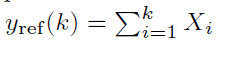# Index exceeds matrix dimenstions error

I am trying to model the below problem using Matlab-CVX:

Here, x, y and u are variables. My code is:

for i = 1: K
cvx_begin
cvx_solver sdpt3
variable u(1,K)
variable x(2,K)
variable y(1,K)

``````    f = 10 * (y(1,i) - y_ref)^2 + (u(1,i))^2;
disp(f);

minimize(f)
subject to
x(1,i+1) == A * x(1,i) + B * u(1,i);
y(1,i) == C * x(1,i);
-1 <= u(1,i) <= 1;

cvx_end
``````

end

However, I am getting error that index exceeds matrix dimensions. I can’t see where it’s causing the problem. Any help is appreciated. Thanks.

You haven’t provided complete reproducible code, to include all MATLAB variables. Nevertheless,

Your error message: On the last time through the for loop, you are constraining x(1,K+1)., which exceeds the declared dimension of x.

Your for loop is in the wrong place. You should have only a single CVX invocation for your problems. You need to sum over all K terms in the objective function (you can use sum, rather than for loop, but for loop could be used and would be valid code).

You need to get all your constraints specified in the single CVX instance. I don’t know why you declare x as 2 by k rather than 1 by k, but other than unnecessarily increasing the size of x, it doesn’t appear to be “wrong”.

1 Like

Hi Mark,
Thanks for replying. I have updated the code and now I dont get the error anymore. However, the result seems wired though. I have declared x(2,K) since in the question it is mentioned that x(k) is a matrix of (2,1) dimension.
Also,Here is the code:

``````clear all;
clc;

has_linprog  = exist( 'linprog' );
has_linprog  = has_linprog == 2 | has_linprog == 3;
rnstate = randn( 'state' ); randn( 'state', 1 );
s_quiet = cvx_quiet(true);
s_pause = cvx_pause(false);
cvx_clear;
echo off

K = 15;
A = [1 0; 0.0833 1];
B = [5; 0.2083];
C = [0 1];
y_ref = 0;
f = 0;

cvx_begin
cvx_solver sdpt3
variable u(1,K)
variable x(2,K+1)
variable y(1,K)

for i = 1: K
y_r = sum(x(:,i));
y_ref = y_ref + y_r;

f1 = 10 * (y(1,i) - y_ref)^2 + (u(1,i))^2;
disp(f);
f = f + f1;
end

minimize(f)
subject to
x(:,i+1) == A * x(:,i) + B * u(1,i);
y(1,i) == C * x(:,i);
-1 <= u(1,i) <= 1;

cvx_end

disp(u)``````

Your code only sets constraints for i = K. That is not what you want.

did not get your point. How can I write the first constraint without using indexing?
If I write the constraints like below:

``````subject to
% x == A * x + B * u;
y == C * x;
-1 <= u <= 1;
``````

Then I get a solution but thats not correct obviously. How can I write the first constraint?

In your code, the constraints are not in a for loop. Therefore, the value of i when the constraint statements appear has the final value from the for loop, which is i = K. That is the only value of i used to specify your constraints. That is not what you want to do.

1 Like

Should I include the `constraints` inside the for loop then and the `minimization()` statement will be after the for loop?

You can do that. It doesn’t matter whether constraints are placed before or after the minimize statement.

Thanks. This is the updated code:

``````cvx_begin
cvx_solver sdpt3
variable u(1,K)
variable x(2,K+1)
variable y(1,K)
f = 0;

for i = 1: K
y_r = sum(x(:,i));
y_ref = y_ref + y_r;
f1 = 10 * (y(1,i) - y_ref)^2 + (u(1,i))^2;
% disp(f);
f = f + f1;

subject to
x(:,i+1) == A * x(:,i) + B * u(1,i);
y(1,i) == C * x(:,i);
-1 <= u(1,i) <= 1;
end

minimize(f)

cvx_end
``````

How can I know that this is right?

I don’t understand what your model actually is. The definition of X, as having an X_1 and X_2 seems confusing. y_r is summing x(1,I) and x(2,i), which I don’t think is what you want.

I suggest you write out everything clearly, then evaluate everything as though they are MATLAB variables, and make sure the correct sequence of calculations is performed. Then declare the relevant variables as CVX variables, and things should work.